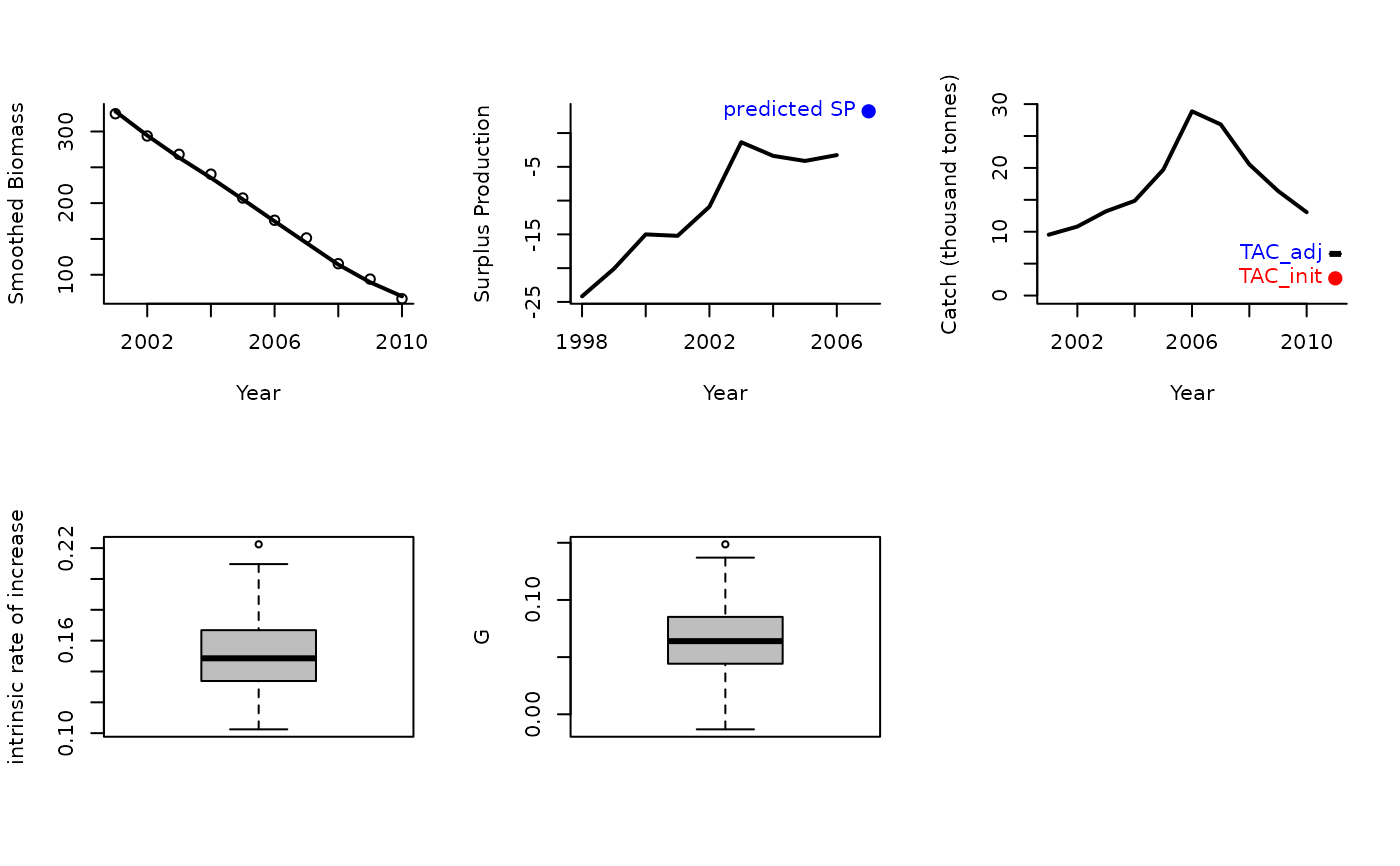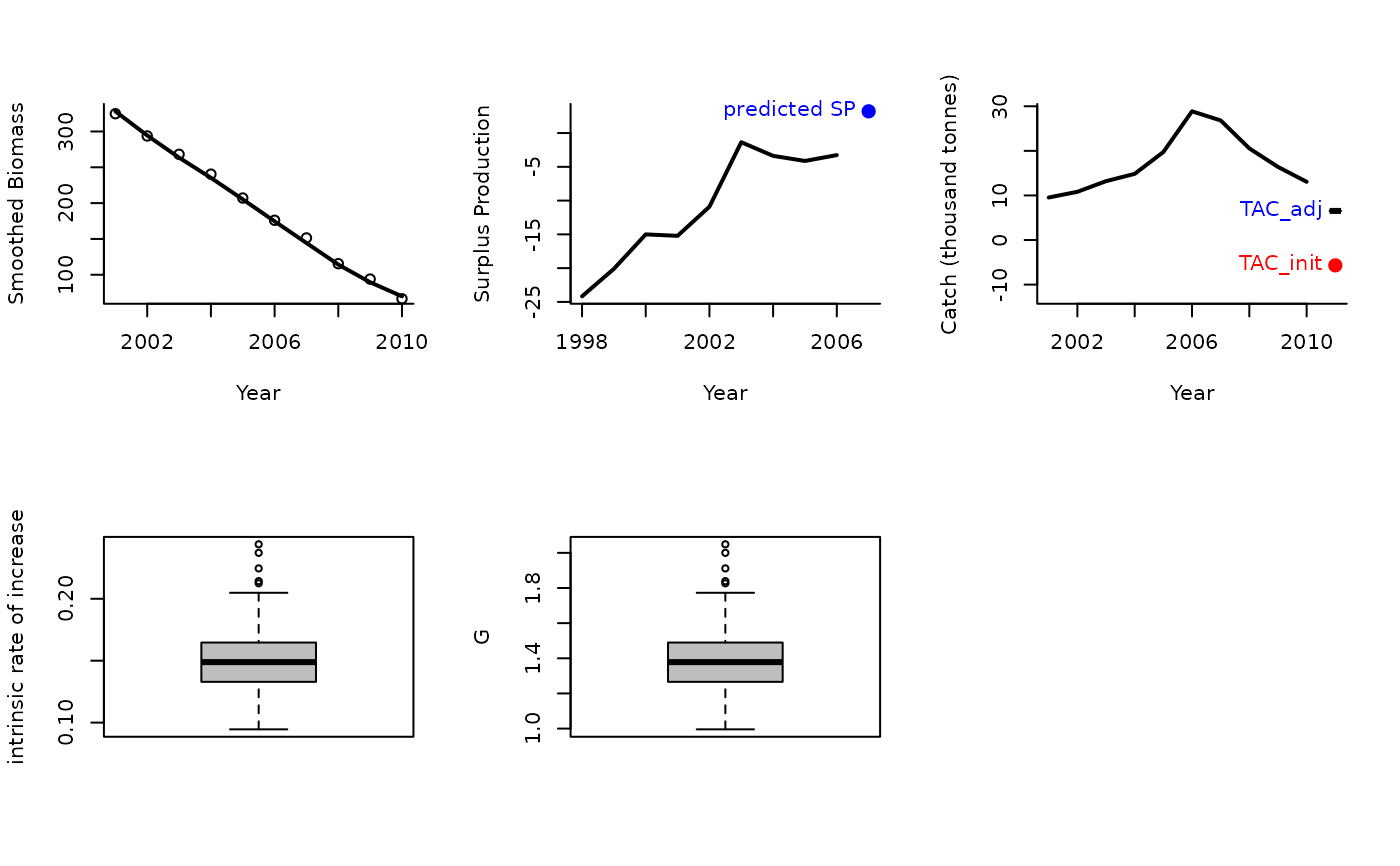An MP proposed by Carl Walters that modifies the TAC according to trends in apparent surplus production that includes information from a demographically derived prior for intrinsic rate of increase

Rcontrol(
x,
Data,
reps = 100,
plot = FALSE,
yrsmth = 10,
gg = 2,
glim = c(0.5, 2)
)

Rcontrol2(
x,
Data,
reps = 100,
plot = FALSE,
yrsmth = 10,
gg = 2,
glim = c(0.5, 2)
)

## Arguments

x

A position in the data object

Data

A data object

reps

The number of stochastic samples of the MP recommendation(s)

plot

Logical. Show the plot?

yrsmth

The number of years for smoothing catch and biomass data

gg

A gain parameters

glim

Limits for the change in TAC among years

## Value

An object of class Rec-class with the TAC slot populated with a numeric vector of length reps

## Details

The TAC is calculated as: $$\textrm{TAC} = \textrm{SP} (1-gG)$$ where $$g$$ is a gain parameter, $$\textrm{SP}$$ is estimated surplus production, and $$G$$ is: For Rcontrol: $$G = r (1-2D)$$ where $$r$$ is the estimated intrinsic rate of increase, and $$D$$ is assumed depletion.

For Rcontrol2: $$G = r - 2bB_\textrm{hist}$$ where $$B_\textrm{hist}$$ is the smoothed biomass overlast yrsmth years and: $$b = \sum{\frac{\textrm{SP}}{B_\textrm{hist}} - r} \frac{\sum{B_\textrm{hist}}}{\sum{B_\textrm{hist}^2}}$$.

The TAC is subject to conditions limit the maximum change from the smoothed catch over the last yrsmth years by the glim argument, e.g, default values of glim = c(0.5, 2) means that maximum decrease in TAC is 50% of average catch and maximum increase is 2 x average catch.

## Functions

• Rcontrol: Base version Rcontrol

• Rcontrol2: This is different from Rcontrol because it includes a quadratic approximation of recent trend in surplus production given biomass

## Required Data

See Data-class for information on the Data object

Rcontrol: Abun, Cat, Dep, FMSY_M, Ind, L50, MaxAge, Mort, Year, steep, vbK, vbLinf, vbt0, wla, wlb

## Rendered Equations

See Online Documentation for correctly rendered equations

Other Surplus production MPs: Fadapt(), SPMSY(), SPSRA(), SPmod(), SPslope()

## Author

C. Walters and T. Carruthers

## Examples

Rcontrol(1, Data=MSEtool::Atlantic_mackerel, plot=TRUE)#> TAC (median)
#>     6.529254
Rcontrol2(1, Data=MSEtool::Atlantic_mackerel, plot=TRUE)#> TAC (median)
#>     6.529254